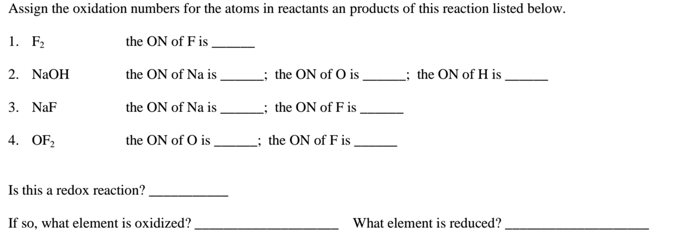# Problem: Fluorine reacts with cold sodium hydroxide solution to produce oxygen difluoride as shown in the following chemical equation 2 F2 (g) + 2 NaOH (aq) → 2 NaF (aq) + H 2O (l) + OF2 (g) Assign the oxidation numbers for the atoms in reactants an products of this reaction listed below.

###### FREE Expert Solution
98% (345 ratings)
###### Problem Details

Fluorine reacts with cold sodium hydroxide solution to produce oxygen difluoride as shown in the following chemical equation

2 F(g) + 2 NaOH (aq) → 2 NaF (aq) + H 2O (l) + OF(g)

Assign the oxidation numbers for the atoms in reactants an products of this reaction listed below.What scientific concept do you need to know in order to solve this problem?

Our tutors have indicated that to solve this problem you will need to apply the Calculate Oxidation Numbers concept. You can view video lessons to learn Calculate Oxidation Numbers. Or if you need more Calculate Oxidation Numbers practice, you can also practice Calculate Oxidation Numbers practice problems.

What is the difficulty of this problem?

Our tutors rated the difficulty ofFluorine reacts with cold sodium hydroxide solution to produ...as medium difficulty.

How long does this problem take to solve?

Our expert Chemistry tutor, Dasha took 3 minutes and 37 seconds to solve this problem. You can follow their steps in the video explanation above.

What professor is this problem relevant for?

Based on our data, we think this problem is relevant for Professor Dixon's class at UCF.